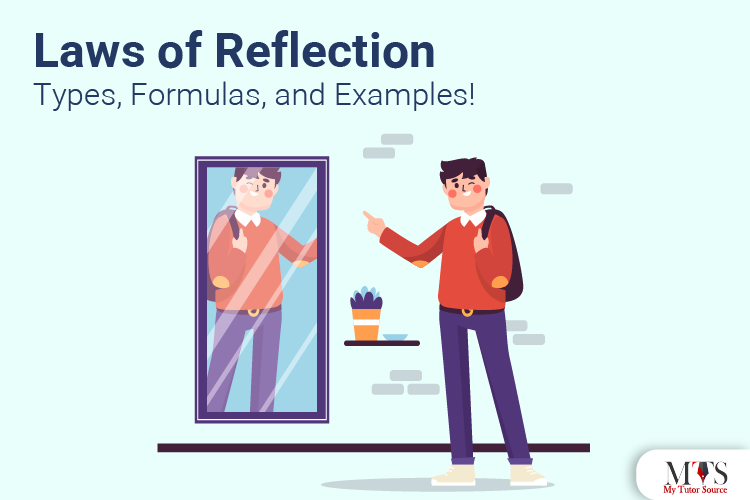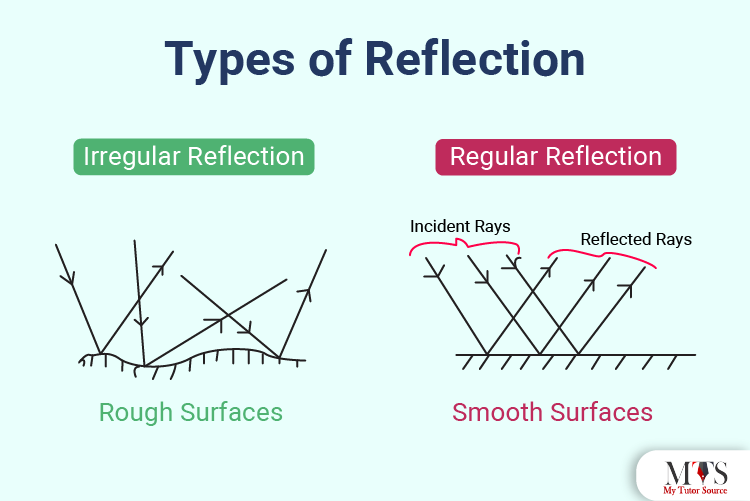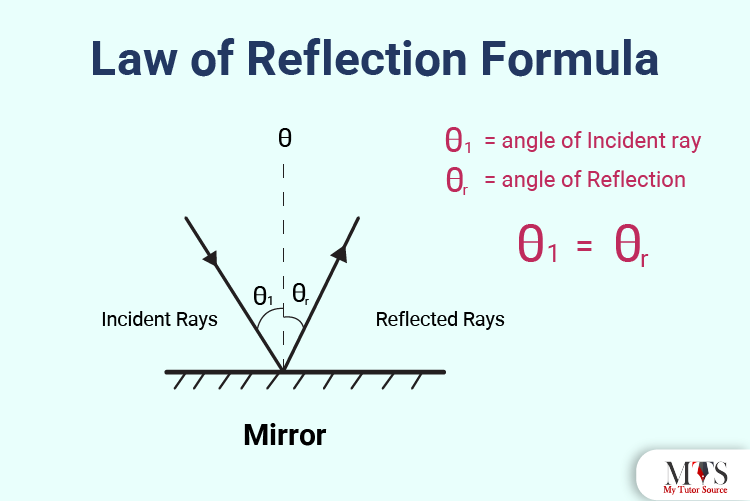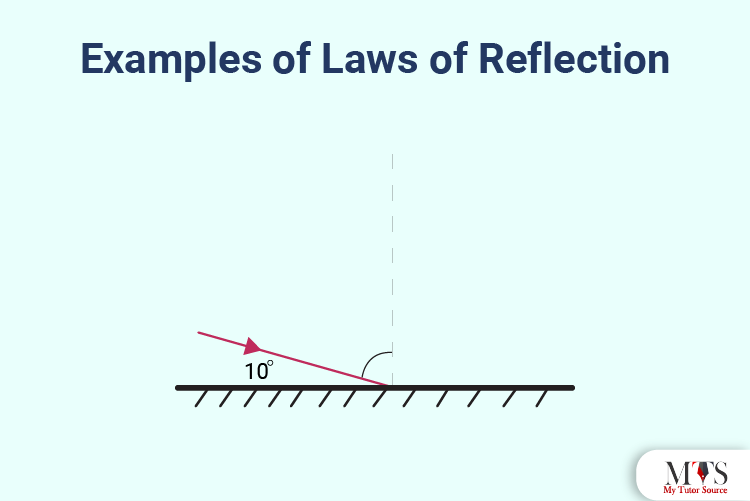# Laws of Reflection: Types, Formulas, and Examples!We see a reflection whenever we stand in front of a mirror or a lake with sunlight glinting on top. Yes, it is common. But whenever someone asks us about the technical details of reflections, we go blank. If you are a student in the same boat trying to learn about Reflection and its laws, you have landed on the right page!

Now we all know that light is a form of energy, right? It goes through various processes like reflection, refraction, interference, and diffraction. However, the most important and common one is Reflection; it is essential to understand how it works, especially if you are a Physics student!

In this post, we will elaborate on the laws of reflection, types of reflection, and their examples in detail. Keep Reading!

## What Is the Law of Reflection?

The law of reflection is basically divided into two more sub-laws. Let's go through those two sub-laws first:

First Law of Reflection: The angle of the reflected ray will always be equal to the angle of the incident ray while reflecting from a smooth surface; concerning the normal to the surface that is to a line perpendicular to the surface at the point of contact.

Second Law of Reflection: The reflected ray is always in the plane defined by the incident ray and the normal to the surface at the point of contact of the incident ray.
With that being said, the scientific definition of the law of reflection states after combining the two sub-laws:

"The principle when the light rays fall on the smooth surface, the angle of reflection is equal to the angle of incidence, also the incident ray, the reflected ray, and the normal to the surface all lie in the same plane."

## What is Reflection of Light?

To understand the law of reflection, it is essential to understand the reflection of light first. Reflection of light is the process in which the light ray falls on a surface and then bounces back.
In complex and scientific words, when one light from a surface falls on another medium, it will bounce back to the same medium, and the angle of incidence is equal to the angle of reflection.
The light reflected from a mirror surface is the perfect example of the reflection of light.

## Types of Reflection

There are mainly two types of reflection:

### Regular Reflection

Regular reflection is produced by a plane mirror with a smooth surface. In easier words, the reflection produced when a light ray strikes a smooth surface is called regular reflection. The images in this type of reflection are usually very clear and visible.
The nature of the regular fraction totally depends on how smooth the surface is. For example, smooth surfaces of silver can reflect the ray of light only in one direction.

### Irregular Reflection

Now that we know that smooth surfaces produce regular reflection, it is evident that irregular reflection will be the opposite. Most of the objects we see daily don't have a smooth surface, right? So, when a light ray strikes a rough surface, the reflected rays are scattered in different directions. This scenario caused by rough surfaces is known as irregular reflection.This image shows what happens when a smooth and rough surface reflects light.

### Difference Between Regular and Irregular Reflection

Now that we know the basic difference between a regular and irregular reflection, here are a few more characteristics that make them different from each other:

 Regular Reflection Irregular Reflection This occurs when rays are reflected from a smooth surface is parallel to parallel incident light rays. This occurs when the reflected rays and parallel incident rays are not parallel with each other. Regular reflection occurs only on smooth surfaces like spoons, mirrors, silver, etc. Irregular reflection only occurs on rough surfaces like curved mirrors, a book, tables, and wood. The image produced by regular reflection is always seen and formed. Irregular reflection only helps to see the objects and forms no images.

### Applications of Regular and Irregular Reflection

We see various applications of regular and irregular reflections in our daily lives. Here are some examples:
1. We can see our image in the mirror through regular reflection of light.
2. The sunlight is turned towards dark places using a shiny surface due to the regular reflection of light.
3. We don't get direct sunlight in our house, but we can still see the objects, right? This happens because of the irregular reflection of light; the light is scattered in different directions.
4. Have you ever seen dust particles in the air when sunlight shines on them? That is also because of irregular reflection of light.

## Law of Reflection Formula,

The formula of the law of reflection, is:
θi = θr
Here,
θi = angle of incidence
θr = angle of reflection### What is the Angle of Reflection?

The angle of reflection of a ray (θr) is the angle you measure from the normal surface to the reflected ray.
You can calculate the angle of reflection and angle of incidence by drawing a normal line perpendicular to the reflecting surface.

## What are Concave Mirrors?

A concave mirror is a type of mirror that gives inverted and real images if the object is not focused, and a virtual and large image if the distance from the pole of the mirror of the object is less than the focal length.
Concave mirrors are usually used in torches, vehicle headlights, and shaving mirrors. The dentist also uses concave mirrors to take a close look at the patient's teeth.

## What are Convex Mirrors?

A convex mirror is the type of mirror that gives a diminished and virtual image when the object is behind the mirror.
Convex mirrors are used in shops to detect burglars, make lenses for glasses, reflect street lights, and magnifying glasses.

## Examples of Laws of Reflection

Below, we are sharing a few solved examples for you to absorb the concept of the law of reflection clearly. Read on!
Example 1: If the light is incident on a flat surface, as shown in the image, and makes an angle of 10o with that surface, what will be the
1. The angle of Incidence?
2. The angle of Reflection?Solution:
1. If the light and surface are making an angle of 10o, then its normal angle would be 80o. Hence, the angle of incidence will be 80o.
2. The law of reflection states that the angle of reflection is always equal to the angle of incidence. Hence, the angle of reflection will be 80o.
Example 2: A ray of light strikes a plane surface at an angle of 50o. What will be its angle of incidence and angle of reflection?
Solution:
We will find the angle of incidence through the following process:
θi = 90o - 50o = 30o.
Now that we know that angle of incidence is equal to the angle of reflection:
θr = 30o,

## Frequently Asked Questions

How many laws of reflection are there in total?
There are a total of 2 laws of reflection.
What is the second law of reflection?
According to the second reflection law, “the incident ray, the normal to the mirror at the point of incidence and the reflected ray, all lie in the same plane.”
Who gave the laws of reflection?
The laws of reflection were introduced by a Greek Mathematician known as Euclid.
Are parallel rays incident on a transparent sphere?
Yes, parallel rays are always incident on a transparent sphere.

### Find Top Tutors in Your AreaWith over 3 years of experience in teaching, Chloe is very deeply connected with the topics that talk about the educational and general aspects of a student's life. Her writing has been very helpful for students to gain a better understanding of their academics and personal well-being. I’m also open to any suggestions that you might have! Please reach out to me at chloedaniel402 [at] gmail.com